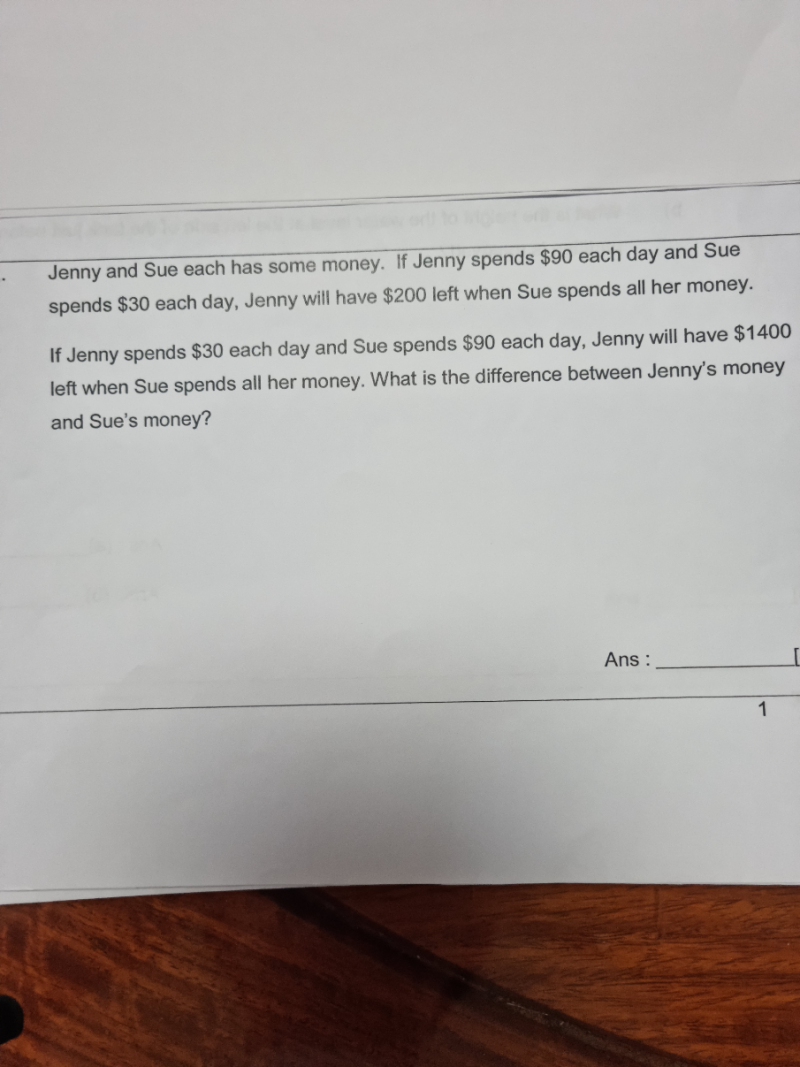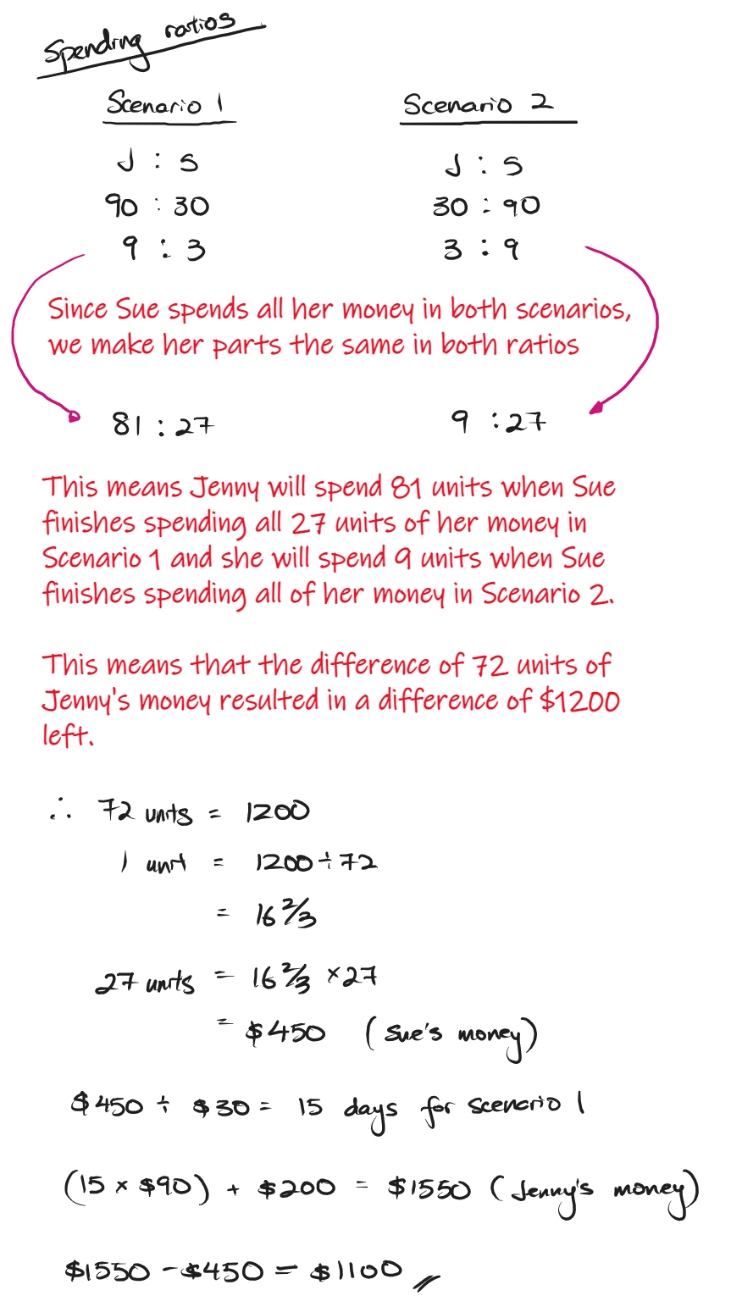# QuestionThank you .

1st scenario               2nd scenario

J      90u  + 200                30p + 1400

S       30u + 0                          90p+0

In both scenarios, the amount remains the same for J and S,

So  90u + 200 = 30p +1400

30u = 90 p => u = 3p

90(3p) + 200 = 30p + 1400

240p = 1200

p = 5

J has 30(5) + 1400 = 1550

S has 90(5)               =   450

Hence difference between their money is (1550-450) = \$1100

0 Replies 1 Like0 Replies 1 Like